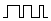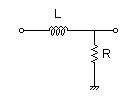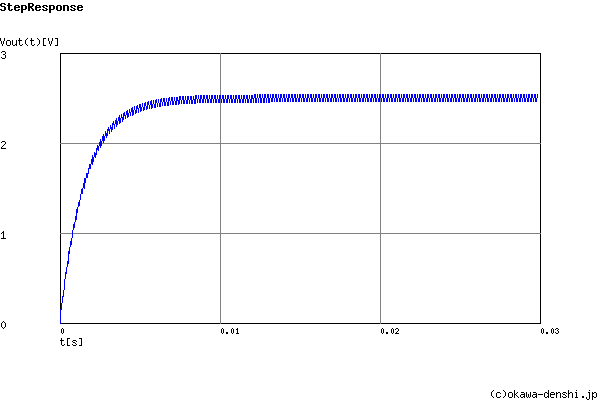# (Sample)LR Low-pass Filter Design for PWM - Result -

Calculated peak-to-peak ripple voltage and settling time at a given PWM frequency and cut-off frequency or values of L and R.

## LR FilterPWM signal →→Vout(s)
(Sample)Transfer Function:
 G(s)= 628.318530718 s+628.318530718

fc = 100[Hz]

g(∞) = 2.5[V]

#### Peak-to-peak ripple voltage

ΔVpk-pk = 0.0785333573362[V](Duty=50%)

#### Settling time 0%→90% (0V→2.25V) (without a ripple)

tr = 0.0036646779944[sec]
fPWM=Hz
Duty Step 0%→[%]

PWM signal voltage:
VL = [V]  VH = [V]

### L and R values of filter | Cut-off frequency

Cut-off frequency fc = [Hz]
L and R values
 R=Ω L=H
p:pico, n:nano, u:micro, k:kilo, M:mega

 Transient analysis